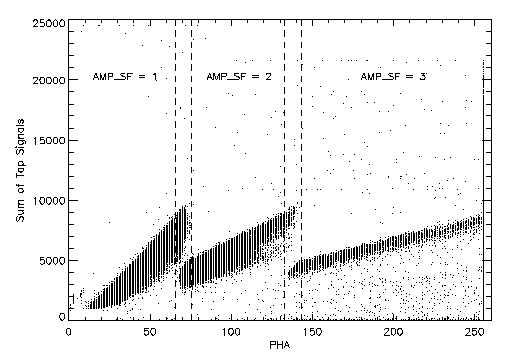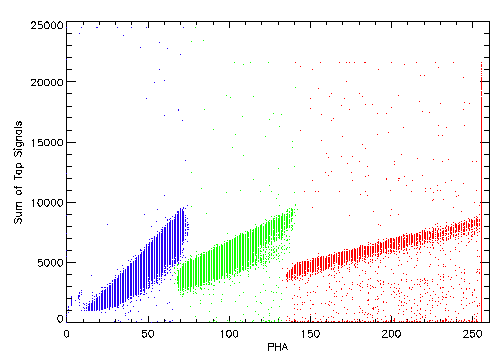# An Improved AMP_SF Correction Scheme

## Introduction

In an earlier memo, I compared two different schemes for correcting the AMP_SF values on an event-by-event basis. Both of the methods did a good job on data from the HRC-I but had problems making good assignments for HRC-S data. Both methods relied on the expectation that the ratio between the sum of the signals on the six CGCD taps and the event pulse-height (PHA) should be close to multiples of 2 once the gain difference between the amplifiers is taken into account. A higher level of "noise" on the tap signals of the HRC-S leads to problems with this assumption. However, revisiting how the range is selected by the hardware leads to yet another AMP_SF correction scheme.

## New Correction Method

The HRC selects the range setting by comparing the output signal from the MCPs to a commanded threshold value. The telemetered PHA value should provide a measure of size of the signal from the MCPs. We should be able to use the PHA value along with knowledge of the commanded range-switch level to determine the correct scale factor. Noise in the relationship between the MCP signal and the PHA value can lead to an ambiguity in what the appropriate AMP_SF value is for a given PHA value. We make the assumption that outside of a specified band around the PHA at the range-switch points, the AMP_SF value is unambiguous. Within the band around the range-switch points the AMP_SF value can only be one of the two surrounding values and the correct one can be selected as the one that gives an answer that is "closest" to the nominal ratio between the sum of the six tap values and the PHA. Figure 1 shows a schematic representation of this method. The PHA values where the AMP_SF assignment can be made unambiguously are labeled. Events with PHA values that are within the two intervals indicated by the vertical dashed lines require an additional test comparing the sum of the six tap signals to PHA to select between the two possible AMP_SF values.Figure 1: Sum of Six Tap Values versus PHA - the bands that define the range-switch regions are indicated by the dashed lines.

### PHA values at Range-switch Point

We can examine data to determine the PHA values at the middle of the range-switching bands. The HRC-I and HRC-S will not necessarily switch at the same PHA value when commanded with the same range-switch level. An additional complication with which we must deal is the fact that the commanded range-switch value has changed for both the HRC-I and HRC-S from the ones used at the start of the mission. Table 1 shows the PHA values for the middle of the range-switch band as observed in data taken on-orbit as a function of commanded range switch level.

Table 1: PHA of Range-switch Points
DetectorRange-switch LevelPHA at 1 to 2PHA at 2 to 3
HRC-I9050.599.0
11564.5126.5
HRC-S9051.099.5
12570.5137.5

Fortunately, the PHA values at the range-switch points appear to be essentially the same, as a function of commanded range-switch level, for the HRC-I and HRC-S. The AMP_SF 1 to 2 PHA value is ~0.57× the commanded range-switch level, while the AMP_SF 2 to 3 PHA value is ~1.10× the commanded range-switch level. For the HRC-I, events within a band of ±2.0 PHA channels around the values in table 1 require the additional check to determine the correct AMP_SF, while for the HRC-S the band must be enlarged to ±5.0 PHA channels.

### Check on Sum of Six Taps vs PHA

When the events lie within the bands of PHA values around the range-switch points an additional check is required to obtain the best AMP_SF value. Within this band we can use the fact that we expect the sum of the six tap signals (suitably normalized) to be the same as the PHA within a factor of two, thus giving the correct AMP_SF value. For each event that is in the band, the absolute value of the difference between the sum of the six taps, scaled by the gain ratio between the taps and the PHA, and the PHA is calculated for the two relevant AMP_SF values. The AMP_SF value that produces the smallest difference is then selected.

### Algorithm

Here is a pseudo-code fragment of the algorithm described above
```# From the event
#   PHA is the event pulse height
#   SUMAMPS is the sum of the 6 CGCD taps (i.e. AU1+AU2+AU3 + AV1+AV2+AV3)
#   AMP_SF re-assigned AMP_SF value
#
# Parameters
#   PHA_1TO2   - middle of the AMP_SF 1 to 2 range-switch band
#   WIDTH_1TO2 - width of the AMP_SF 1 to 2 range-switch band
#   PHA_2TO3   - middle of the AMP_SF 2 to 3 range-switch band
#   WIDTH_2TO3 - width of the AMP_SF 2 to 3 range-switch band
#   GAIN       - relative gain between SUMAMPS and PHA

if (PHA < (PHA_1TO2 - WITDH_1TO2))
AMP_SF = 1
else if (PHA < (PHA_1TO2 + WITDH_1TO2))
AMP_SF = 1
diff1 = abs(PHA - SUMAMPS/GAIN)
diff2 = abs(PHA - 2*SUMAMPS/GAIN)
if (diff2 < diff1) AMP_SF = 2
else if (PHA < (PHA_2TO3 - WITDH_2TO3))
AMP_SF = 2
else if (PHA < (PHA_2TO3 + WITDH_2TO3))
AMP_SF = 2
diff2 = abs(PHA - 2*SUMAMPS/GAIN)
diff3 = abs(PHA - 4*SUMAMPS/GAIN)
if (diff3 < diff2) AMP_SF = 3
else
AMP_SF = 3
endif

```

Figure 2 shows the result of applying this method to some HRC-S data. The points are color-coded based on their assigned AMP_SF values. There is no evidence for the mis-assignments that were made by either of the two methods presented in the earlier memos.Figure 2: Sum of Six Tap Values versus PHA color-coded by the corrected AMP_SF value. Blue: AMP_SF=1, Green: AMP_SF=2, and Red: AMP_SF=3.

## Conclusion

I have devised yet another algorithm for correcting the AMP_SF values of HRC events. While it is somewhat more complicated, it does a better job at correcting the AMP_SF values on the HRC-S than either of the two methods discussed previously. I recommend that this method be adopted for use in a revised version of hrc_process_events.